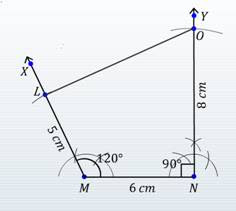## 12 September 2014

### Practical Geometry : CBSE / NCERT Revision Notes

Different quadrilaterals can be identify the based on the properties of sides, diagonals and angles.
Quadrilaterals are made up of ten parts. However, to construct them, do not need to know the measurements of all of them.
In case of special quadrilaterals, like the rectangle, just two measurements, the lengths of its adjacent sides are enough to construct it.
A kite can be constructed if the lengths of its distinct adjacent sides and one diagonal are known. Similarly, a square can be constructed with just the length of its side, while a rhombus can be constructed when the lengths of its diagonals are known.

### Steps to Construct a Rectangle:

Step 1: Draw a side of given length (say) CL
Step 2: Draw side LU (say) of given length perpendicular to CL at L.
Step 3: Draw side CE (say) of length equal to LU and perpendicular to CL at C.
Step 4: Draw side UE.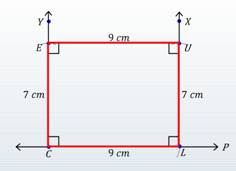### Steps to Construct a Kite:

Step 1: Draw diagonal (say) AY and its perpendicular bisector.
Step 2: Draw sides say AL and AZ of given length.
Step 3: Draw sides LY and YZ.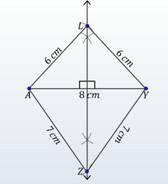### Constructing a Quadrilateral when four sides and one of its diagonals are given.

Step 1: Construct a triangle ABD (say).
Step 2: Find point C opposite to the vertex A as follows. With B as the centre and given radius, draw an arc on the other side of BD. Similarly with D as the centre and given radius, draw another arc intersecting the previous arc. The point of intersection of these arcs is marked as C.
Step 3: join points B and C, and D and C.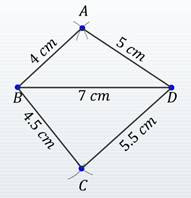### Constructing a quadrilateral when lengths of its three sides and two diagonals are given.

Step 1: Construct a triangle PQR (say).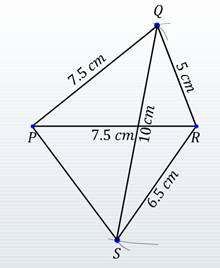### Constructing a quadrilateral when lengths of its adjacent sides and three angles are given.

Step 1: Draw a line segment of EF (say) of given length.
Step 2: Construct a given angle at E.
Step 3: Construct a given angle at F.
Step 4: Locate point G.
Step 5: Locate point H.### Constructing a quadrilateral when lengths of its three sides and two included angles.

Step 1: Draw a line segment MN (say) of given length.
Step 2: Construct a given angle at M.
Step 3: Construct an angle 90° at N.
Step 4: Locate vertices L and O.
Step 5: Join L and O.